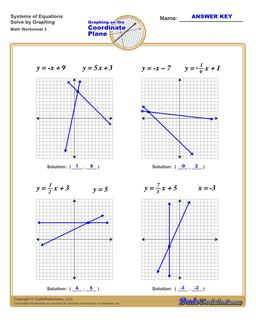PLEASE GO BACK AND USE THE BIG BLUE 'PRINT' BUTTON ON THE PAGE TO PRINT THE WORKSHEET CORRECTLY!Sorry for the trouble! The browser won't print the embedded worksheet PDF directly using the normal 'Print' command in the file menu, so you need to click the big 'Print' button to send just the worksheet and not the surrounding page to the printer.

# Math Worksheets: Linear Equations: Linear Equations: Graphing Systems of Equations (Third Worksheet)## Graphing Systems of Equations (Third Worksheet)

Property Value
DescriptionGraphing Systems of Equations: The worksheets on this page have four coordinate planes and systems equations in slope intercept form that students graph to solve, and includes an answer key showing the correct graph. (Third Worksheet)
Resource TypeWorksheet